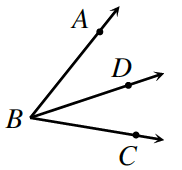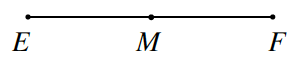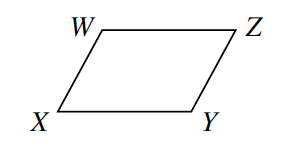### Home > INT2 > Chapter 6 > Lesson 6.1.2 > Problem6-24

6-24.

Use the information given for each diagram below to solve for $x$. Show all work.

1. $\overrightarrow{ B D }$ bisects $∠ABC$. (Remember that an angle bisector divides the angle into two congruent angles.) If $m∠ABD = 5x - 10^\circ$ and $m∠ABC = 65^\circ$, solve for $x$.

$2(5x - 10^\circ) = 65^\circ$1. Point $M$ is a midpoint of $\overline{EF}$. If $EM = 4x - 2$ and $MF = 3x + 9$, solve for $x$.

If $M$ is the midpoint, how are $\overline{EM}$ and $\overline{MF}$ related?1. $WXYZ$ at right is a parallelogram. If $m∠W = 9x - 3^\circ$ and $m∠Z = 3x + 15^\circ$, solve for $x$.

How are the angles of the parallelogram related?